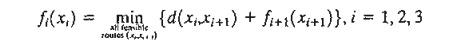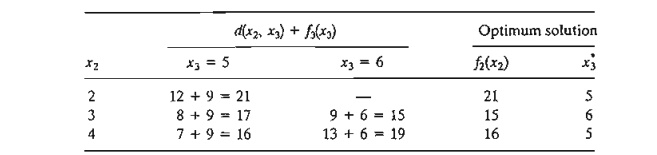Home | | Resource Management Techniques | Forward and Backward Recursion- Dynamic Programming

# Forward and Backward Recursion- Dynamic Programming

Both the forward and backward recursions yield the same solution. Although the forward procedure appears more logical, DP literature invariably uses backward recursion.

FORWARD AND BACKWARD RECURSION

Example 10.1-1 uses forward recursion in which the computations proceed from stage 1 to stage 3. The same example can be solved by backward recursion, starting at stage 3 and ending at stage l.

Both the forward and backward recursions yield the same solution. Although the forward procedure appears more logical, DP literature invariably uses backward recursion. The reason for this preference is that, in general, backward recursion may be more efficient computationally. We will demonstrate the use of backward recursion by applying it to Example 10.1-1. The demonstration will also provide the opportunity to present the DP computations in a compact tabular form.

Example 10.2-1

The backward recursive equation for Example 10.2-1 iswhere f4(x4)  = 0 for x4  = 7. The associated order of computations is f3 - > f2- >f1.

Stage 3. Because node 7 (x4 = 7) is connected to nodes 5 and 6 (x3 = 5 and 6) with exactly one route each, there are no alternatives to choose from, and stage 3 results can be summarized asStage 2. Route (2, 6) is blocked because it does not exist. Given f3(x3) from stage 3, we can compare the feasible alternatives as shown in the following tableau:The optimum solution of stage 2 reads as follows: If you are in cities 2 or 4, the shortest route passes through city 5, and if you are in city 3, the shortest route passes through city 6.

Stage 1. From node 1, we have three alternative routes: (1,2), (1, 3), and (1,4). Using h(X2) from stage 2. we can compute the following tableau.The optimum solution at stage 1 shows that city 1 is linked to city 4. Next, the optimum solution at stage 2 links city 4 to city 5. Finally. the optimum solution at stage 3 connects city 5 to city 7. Thus, the complete route is given as 1 -> 4 -> 5 -> 7. and the associated distance is 21 miles.

PROBLEM SET 10.2A

For Problem 1, Set 10.1a, develop the backward recursive equation, and use it to find the optimum solution.2. For Problem 2, Set l0..la, develop the backward recursive equation, and use it to find the optimum solution.

*3. For the network in Figure 10.3, it is desired to determine the shortest route between cities 1 to 7. Define the stages and the states using backward recursion, and then solve the problem.

Study Material, Lecturing Notes, Assignment, Reference, Wiki description explanation, brief detail
Operations Research: An Introduction : Deterministic Dynamic Programming : Forward and Backward Recursion- Dynamic Programming |